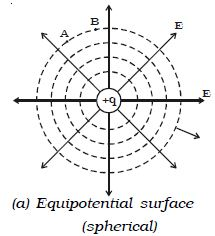# Equipotential Surface

If all the points of a surface are at the same electric potential, then the surface is called an equipotential surface.

(i) In case of an isolated point charge, all points equidistant from the charge are at same potential. Thus, equipotential surfaces in this case will be a series of concentric spheres with the point charge as their centre (Fig: a). The potential, will however be different for different spheres.If the charge is to be moved between any two points on an equipotential surface through any path, the work done is zero. This is because the potential difference between two points A and B is defined as VB – VA = WAB/q

If VA = VB then WAB = 0. Hence the electric field lines must be normal to an equipotential surface.

(ii) In case of uniform field, equipotential surfaces are the parallel planes with their surfaces perpendicular to the lines of force as shown in Fig b.